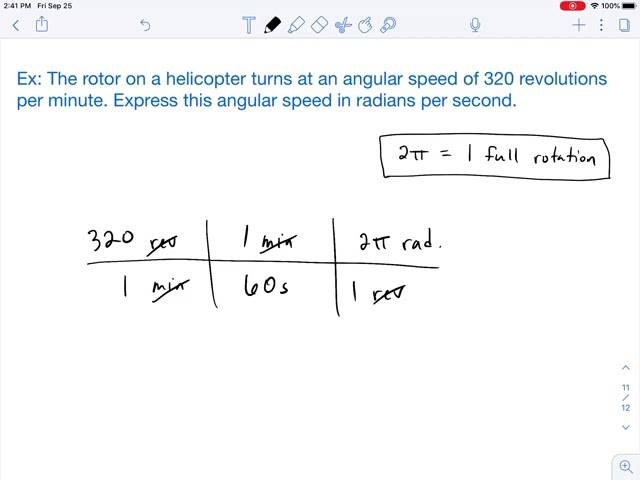• ChiralSuperfields
In summary, the equation allows for multiplying 100,000 t by 1000kg/t to get 10E8 kg. This is incorrect because 0 kg = 0 t.f

#### ChiralSuperfields

Homework Statement
Relevant Equations
Suppose I want to convert 100,000 metric Tonnes to kilograms, then I would perform, a unit cancellation:

Given that 1 t = 1000 kg

##100,000 t \times \frac{1000~kg}{1 t} = 1 \times 10^8 kg##, however, why are we allowed to multiply the 100,000 by that?

My reasoning is,
##1 t = 1000 kg##
##1 = \frac{1000 kg}{1 t}## therefore multiplying by ##100,000 t## by ##\frac{1000 kg}{1 t}## is the same as multiplying by 1

Does someone please know whether my reasoning is correct?

Many thanks!

Last edited:
Yes

••SammyS and ChiralSuperfields
Yes

Suppose I want to convert 100,000 metric Tonnes to kilograms, then I would perform, a unit cancellation:

Given that 1 t = 1000 kg

##100,000 t \times \frac{1000~kg}{1 t} = 1 \times 10^8 kg##, however, why are we allowed to multiply the 100,000 by that?

My reasoning is,
##1 t = 1000 kg##
##1 = \frac{1000 kg}{1 t}## therefore multiplying by ##100,000 t## by ##\frac{1000 kg}{1 t}## is the same as multiplying by 1

Does someone please know whether my reasoning is correct?
No, it is not. Treat the dimensions in the equation as you would unknown variables.

•ChiralSuperfields
No, it is not. Treat the dimensions in the equation as you would unknown variables.
You need to expand on that.

•hutchphd, DaveE and ChiralSuperfields
##100,000t \times 1 = 100,000t## which is incorrect not the answer to the question.

##\frac t t=1## as a next step yields the correct answer.

Last edited:
•ChiralSuperfields
No, it is not. Treat the dimensions in the equation as you would unknown variables.
How is that different?

I guess you mean something like this?
##ax = by \Rightarrow x = \frac{b}{a} y##
then substitute to get ## cx = c (\frac{b}{a} y) = (c \frac{b}{a}) y ##
where ## a,b,c ## are constants and ## x, y ## are units (variables?)

##ax = by \Rightarrow 1 = \frac{by}{ax} ##
## cx = cx (1) = cx (\frac{by}{ax})= (c \frac{b}{a}) y ## as others suggested.

I feel like I'm missing your point here.

•hutchphd, ChiralSuperfields and Frabjous
You can multiply 100,000t by 1 and you do indeed get 100,000t. So what? That not what is sought. What is sought is to get a final answer in kg, not in t. To do that you need to multiply 100,000t by 1000Kg/t to get 10E8Kg. Units matter.

•hutchphd and ChiralSuperfields
My point is the OP used "reasoning" which, while useful in creating or validating a conversion factor, doesn't solve the equation by itself whereas, after including it in the calculation, simple cancellation does :
##100,000 \cancel t \times \frac{1,000kg}{\cancel t} = 100,000,000kg##

Last edited:
•ChiralSuperfields
##1 = \frac{1000 kg}{1 t}##
I believe that such equation is not correct.
##1000~kg/t## is simply a rate, a proportion.
It is used only because it is useful in mathematical operations, where it can be cancelled to obtain the desired units.

IMHO, it is not different from ##3600~s/h## or ##1000~km/m##

•ChiralSuperfields
I believe that such equation is not correct.
##1000~kg/t## is simply a rate, a proportion.
It is used only because it is useful in mathematical operations, where it can be cancelled to obtain the desired units.

IMHO, it is not different from ##3600~s/h## or ##1000~km/m##
Is there a situation where assuming the equation is correct will get you in trouble?
I agee that it is not different from 3600s/h or 1000m/km.

•ChiralSuperfields
Is there a situation where assuming the equation is correct will get you in trouble?
I agee that it is not different from 3600s/h or 1000m/km.
It is not incorrect if used as a conversion factor ; but the OP specifically asked "Are we allowed to multiply like that"... then proceeded to not bother, or at least not show the bother.

•ChiralSuperfields
It is not incorrect if used as a conversion factor ; but the OP specifically asked "Are we allowed to multiply like that"... then proceeded to not bother, or at least not show the bother.
I am looking for the substantive reason that you believe it is sometimes wrong.

Last edited:
•ChiralSuperfields
I am looking for the substantive reason that you believe it is wrong.
That I believe what is wrong ? That ##100,000t = 100,000t## is not a useful answer for "convert from tonnes to kg's" ?

•ChiralSuperfields
Is there a situation where assuming the equation is correct will get you in trouble?
I agree that it is not different from 3600s/h or 1000m/km.
No, unless it is taken out of context.
It may be mathematically correct, but I don't know enough to see any value in something like
##1=1~kilo-banana/1000~bananas##.•ChiralSuperfields and hmmm27
No, unless it is taken out of context.
It may be mathematically correct, but I don't know enough to see any value in something like
##1=1~kilo-banana/1000~bananas##.
Obviously you have never studied economics on the Planet of the Apes.Low utility situations do not invalidate a concept.

There are known pitfalls when dimensionality comes into play. For example, the addition of non-dimensional numbers or the dimensional equivalence of torque and energy. We struggle through.

Last edited:
•ChiralSuperfields, DaveE and SammyS
•Lnewqban